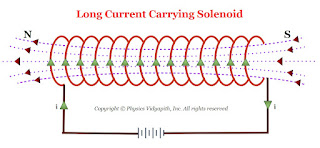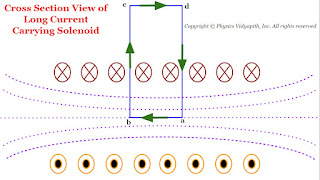### Principle, Construction and Working of Current Carrying Solenoid

Current Carrying Solenoid:

The Solenoid is an artificial magnet which is used for different purposes.

Principle of Solenoid:

The principle of the solenoid is based on the "Ampere Circuital Law" and its magnetic field is raised due to the current carrying a circular loop.

Construction of Solenoid:

The current carrying solenoid is consist of insulated cylindrical material and conducting wire. The conducting wire like copper is wrapped closely around the insulated cylindrical material ( like cardboard, clay, or plastic). The end faces of the conducting wire are connected to the battery.Long Current Carrying Solenoid

Working:

When the electric current flow in the solenoid then a field (i.e. Magnetic field) is produced around and within the current carrying solenoid. This magnetic field is produced in solenoid due to circular loops of the solenoid and the direction of the magnetic field is depend upon the direction of the electric current flow in the circular loop. The magnetic field within the current carrying solenoid is uniform and parallel to the axis of the solenoid.

Derivation of the magnetic field due to long current carrying Solenoid:Cross Section View of the long Current Carrying Solenoid
Let us consider a very long current carrying solenoid of length $l$ in which $i$ electric current is flowing. Here its diameter is very less as compared to the length of the solenoid.

Now take a closed rectangular path $abcd$ in which the side $ab$ is parallel to the axis of the solenoid and sides $bc$ and $da$ are very long so that the side $cd$ is far from the solenoid and the magnetic field at this side is negligibly small.

Now apply Ampere's circuital law to the rectangular path $abcd$

$\oint \overrightarrow{B}. \overrightarrow{dl}=\mu_{\circ} i' \qquad(1)$

Where $i'$ is the current enclosed by the rectangle.

Let $n$ is the number of turns per unit length of the solenoid. So the number of turns in a length $x$ is = $nx$

The current in each turn is $i$ then the net current $(i')$ enclosed by the rectangle $abcd$ is $nxi$ i.e

$i'=nxi$

Now substitute the value of $i'$ in above equation $(1)$

$\oint \overrightarrow{B}. \overrightarrow{dl}=\mu_{\circ} nxi \qquad(2)$

Now expand the Ampere circuital law for closed rectangular $abcd$-

 $\oint \overrightarrow{B}.\overrightarrow{dl}=\int_{a}^{b} \overrightarrow{B}.\overrightarrow{dl}+ \int_{b}^{c} \overrightarrow{B}.\overrightarrow{dl} + \int_{c}^{d} \overrightarrow{B}.\overrightarrow{dl} + \int_{d}^{a} \overrightarrow{B}.\overrightarrow{dl} \qquad(3)$

In above equation the term:

$\int_{b}^{c} \overrightarrow{B}.\overrightarrow{dl}= \int_{d}^{a} \overrightarrow{B}.\overrightarrow{dl}=0$

The above term is zero because along $bc$ and $da$ the magnetic field $\overrightarrow{B}$ and length element $\overrightarrow{dl}$ are perpendicular to each other.

$\int_{c}^{d} \overrightarrow{B}.\overrightarrow{dl}=0$

The above term is zero because the magnetic field $\overrightarrow{B}$ outside the solenoid is negligible due long length of the solenoid.

The above equation $(3)$ can be written by applying the above condition-

$\oint \overrightarrow{B}.\overrightarrow{dl}=\int_{a}^{b} \overrightarrow{B}.\overrightarrow{dl}$

$\oint \overrightarrow{B}.\overrightarrow{dl}= \overrightarrow{B}\int_{a}^{b} \overrightarrow{dl}$

$\oint \overrightarrow{B}.\overrightarrow{dl}= B x \qquad(4)$

Where $x$- Length of $ab$

From equation $(2)$ and equation $(4)$, we get

$Bx=\mu_{\circ}nxl$

 $B=\mu_{\circ}nl$